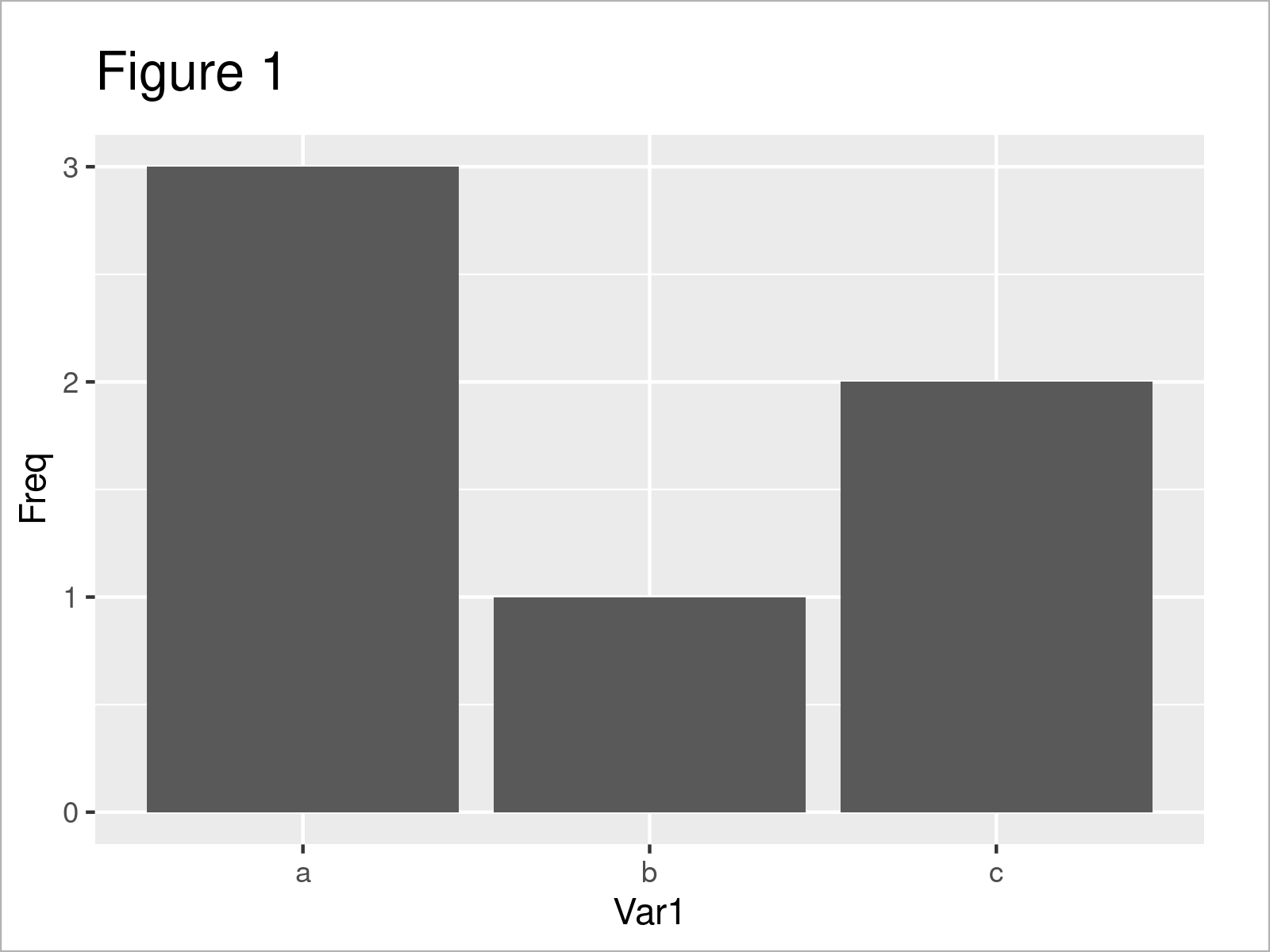# Visualize Table Object in Graphic in R (Example)

In this R tutorial you’ll learn how to draw a plot of a table object.

The tutorial consists of these contents:

Let’s dive right in:

## Creating Example Data

I use the following data as basement for this R tutorial.

```my_tab <- table(c("a", "a", "b", "a", "c", "c"))  # Create example table
my_tab                                            # Print example table
# a b c
# 3 1 2```

The previous output of the RStudio console shows the structure of our exemplifying data: A data object with the table class.

## Example: Draw Barchart of Table Using ggplot2 Package

This example demonstrates how to draw a table object in a plot.

For this, we’ll use the functions of the ggplot2 package. In order to use the functions of the ggplot2 package, we first need to install and load ggplot2:

```install.packages("ggplot2")                       # Install ggplot2 package

Next, we have to convert our table to the data.frame class using the as.data.frame function:

```my_data <- as.data.frame(my_tab)                  # Convert table to data.frame
my_data                                           # Print data frame```In the next step, we can use this data frame to draw a ggplot2 barplot of our data:

```ggplot(my_data,                                   # Draw barchart of table
aes(x = Var1,
y = Freq)) +
geom_bar(stat = "identity")```As shown in Figure 1, we have plotted a ggplot2 barchart showing the values of our table in separate bars by running the previous syntax.

## Video, Further Resources & Summary

If you need further info on the R programming code of this tutorial, you might want to watch the following video that I have published on my YouTube channel. I illustrate the contents of this article in the video.

Please accept YouTube cookies to play this video. By accepting you will be accessing content from YouTube, a service provided by an external third party.If you accept this notice, your choice will be saved and the page will refresh.

Furthermore, you might want to read some of the related articles on my website.

To summarize: In this tutorial you have learned how to create a plot based on a table object in R. Let me know in the comments section, in case you have any additional questions.

Subscribe to the Statistics Globe Newsletter